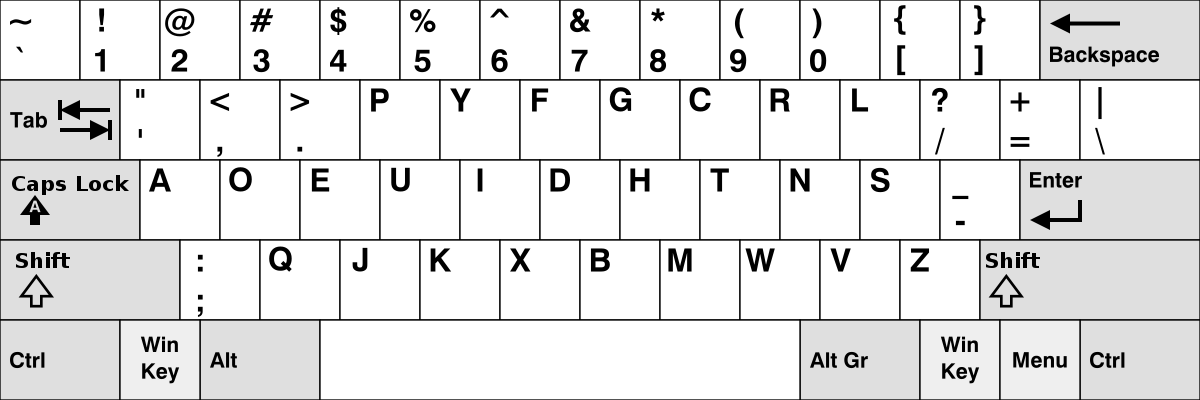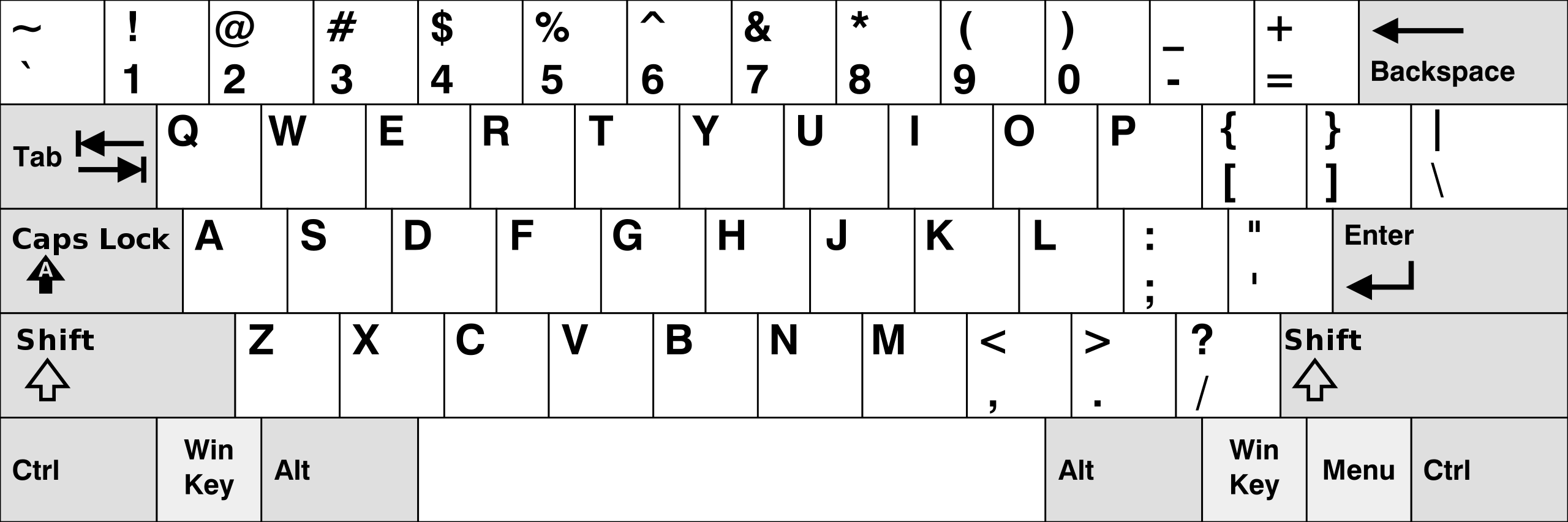# The QWERTY-Dvorak Permutation

By Monte Fischer

Last updated: 21 Mar 2021

I use the Dvorak keyboard layout instead of the standard QWERTY. I’m non-zealous about this choice – it’s simply what I learned to type on in ninth grade, and has been getting in the way ever since. By now, QWERTY feels horribly awkward to type with and I figure that I’m stuck with the Dvorak choice.

#### DvorakWhen people look at my keyboard, they see what any other keyboard would look like. I don’t remap my keys at the hardware level. Instead, I remap them at the software level, and rely completely on muscle memory for typing. Very hard to hunt and peck when the keys are not labeled correctly! This has the amusing side-effect that anyone who tries to type on my computer is immediately bewildered. People make some funny guesses about how the keys have been remapped – a common first guess is that everything is shifted over one. No, no. There is no rhyme or reason to the mapping itself, just one arbitrary permutation of keys to another.

Wait a minute. Permutations?

This sounds like group theory! I didn’t major in mathematics for nothing, after all. Let’s suppose that the QWERTY layout is “standard order”, and investigate the Dvorak permutation that maps each key of the standard QWERTY layout to its respective Dvorak key. There are 26 letters and 9 punctuation characters that get switched around (ignoring the shift key) for a total of 35 symbols. We’ll call this permutation $$\pi$$.

#### Qwerty#### DvorakAs anyone with some group theory under their belt can tell you, this mapping is a permutation of the set of 35 elements. Such a permutation can be decomposed into a product of cyclic permutations, for example Qwerty w goes to Dvorak , and as it happens Qwerty , goes to Dvorak w. This is a small, length 2 cyclic permutation — but they can be longer, of the general form $$A \to B \to C \to \dots \to A$$. Group theory says that any permutation you can think of can be decomposed into combination, or product, of such cycles, where no two cycles share elements (i.e. they are disjoint cycles).

A quick Python script can compute the cycles for us (dv_map is a dictionary encoding the action of $$\pi$$ on each of the 35 characters).

def find_cyclic_decomposition():
letters = list(dv_map.keys())
cycles = []
while len(letters) > 0:
first_letter = letters
curr_letter = dv_map[letters]
this_cycle = [first_letter]
while curr_letter != first_letter:
this_cycle.append(curr_letter)
curr_letter = dv_map[curr_letter]
cycles.append(this_cycle)
for element in this_cycle:
letters.remove(element)
return cycles


The cyclic decomposition of $$\pi$$ thus computed is as follows (the parenthesis are included to tell you where the cycle begins and ends; parenthesis characters themselves are not in different places between QWERTY and Dvorak):

$$\pi =$$

( - [ / z ; s o r p l n b x q ' )
* ( e . v k t y f u g i c j h d )
* ( = ] )
* ( w , )
* ( a )
* ( m )


Very interesting! One cycle of order 15, one of order 14, two of order 2, and two singletons.

Suppose that I had a hardware Dvorak keyboard, and also applied the software mapping. Then I would have mapped the QWERTY keys to Dvorak in hardware, and then again in software. This is equivalent to applying the permutation $$\pi^2$$. I wonder how many times we would have to apply the QWERTY-Dvorak transformation until we got back to the QWERTY layout? In group-theoretic terms, we’re asking for the order of $$\pi$$ in $$S_{35}$$. This is simply the least common multiple of the lengths of all the cycles in the cyclic decomposition: 210.